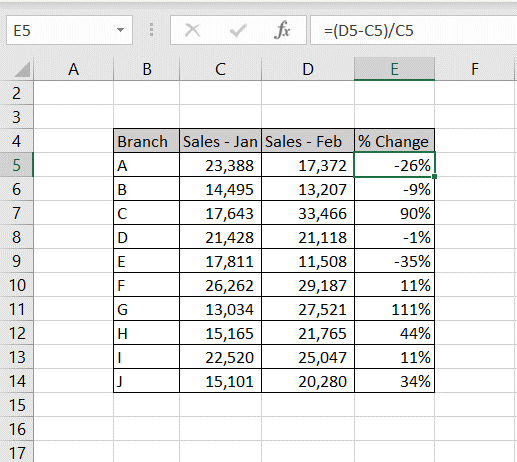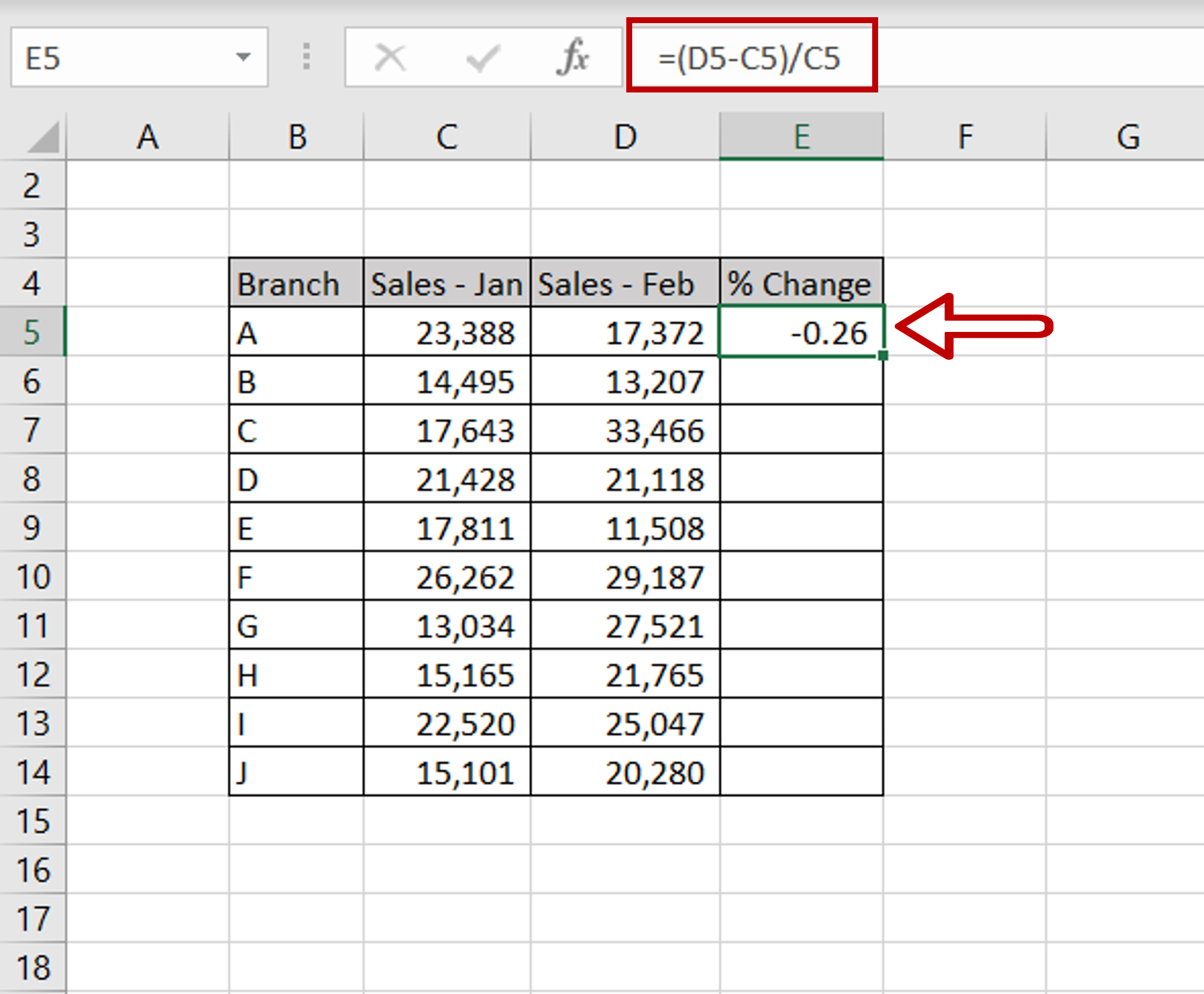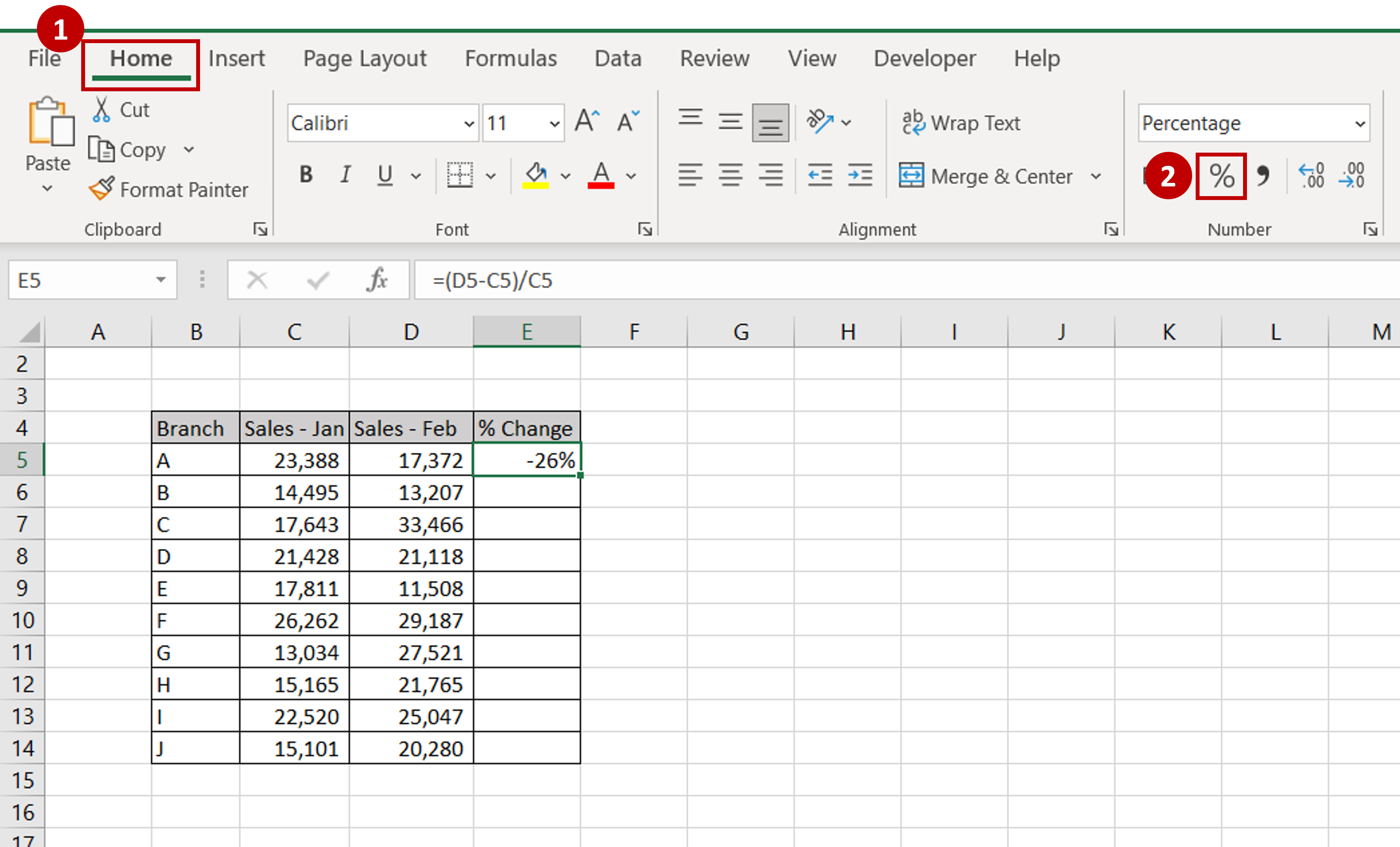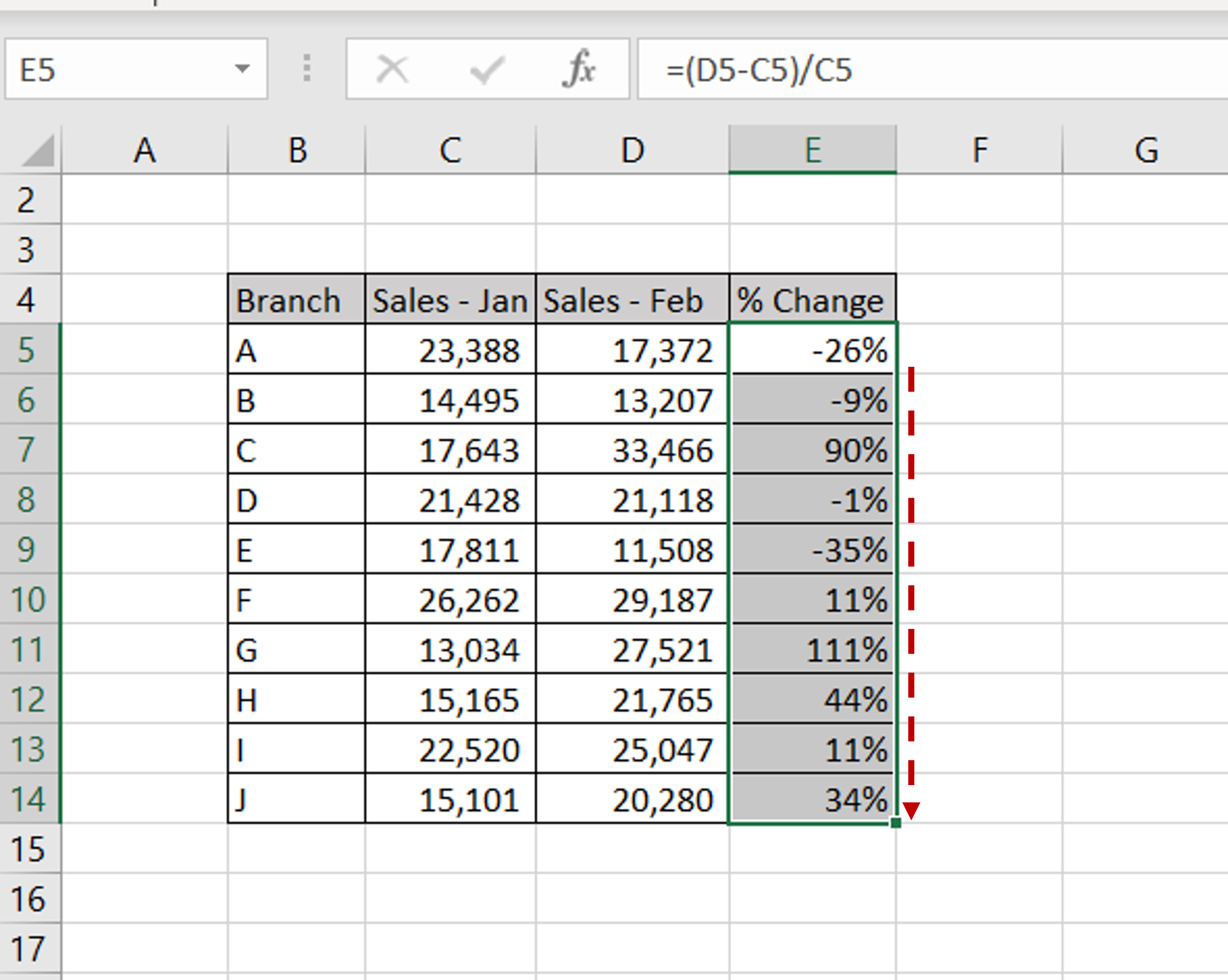# How to calculate variance percentage in Excel

You can watch a video tutorial here.Excel is frequently used for calculations and supports all basic mathematical operations. The variance percentage is the percentage by which one number has changed in a given period. If the change is expressed as a variance percentage, it is easy to compare variances in different periods. In this example, we will see the variance percentage between 2 months for sales. In Excel, the variance percentage can be calculated using the following formula:

((New number – Old number)/Old number)*100

### Step 1 – Divide the difference in numbers by the first number– In the destination cell type the formula using cell references:
=(Sales Feb – Sales Jan)/ Sales Jan

### Step 2 – Format the number as a percentage– Select the cell with the value
– Go to Home > Number
– Click on the percentage sign (%)
OR
Open the Format Cells window (Home > Number and click on the arrow to expand the menu OR Right-click and select Format Cells  from the context menu OR Go to Home > Cells > Format > Format Cells) and select Number > Percentage and click OK

### Step 3 – Copy the formula– Using the fill handle from the first cell, drag the formula to the remaining cells
OR
a) Select the cell with the formula and press Ctrl+C or choose Copy from the context menu (right-click)
b) Select the rest of the cells in the column and press Ctrl+V or choose Paste from the context menu (right-click)